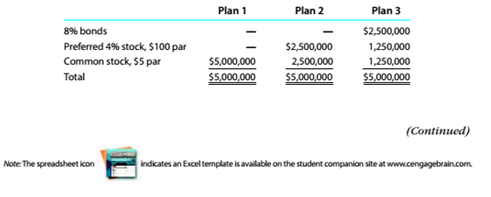Chapter 8, Problem 8.1.1P

Chapter
Section
Textbook Problem

Effect of financing on earnings per shareThree different plans for financing a $5,000,000 corporation are under consideration hy its organizers. I'nder each of the following plans, the securities will be issued at their par or face amount, and the income, tax rate is estimated at 40% of incomeDetermine for each plan the earnings per share of common stock, assuming that the income before bond interest and income tax is$1,000,000.

To determine

Concept Introduction:

Basic Earnings per share:

The Basic Earnings per share is the amount of net income earned by each common share outstanding. The Earnings per share calculated by with help of following formula:

Basic Earnings per share=Net Income - Preferred DividendWeighted Average Common Shares Outstanding

Net Income available to common stockholder = Net income  Preferred Dividend

To Calculate:

The earnings per share for each plan

Explanation

The earnings per share for each plan are calculated as follows:

 Plan 1 Plan 2 Plan 3 Income before interest and tax (A) $1,000,000$ 1,000,000 $1,000,000 Interest on Bonds (B)$ - $-$ 200,000 (2500000*8%) Income before tax (C) = (A-B) $1,000,000$ 1,000,000 $800,000 Income Tax (D) = (C*40%)$ 400,000 \$ 4...

Still sussing out bartleby?

Check out a sample textbook solution.

See a sample solution

The Solution to Your Study Problems

Bartleby provides explanations to thousands of textbook problems written by our experts, many with advanced degrees!

Get Started

Describe four possible types of comparisons that may be made in financial statement analysis.

College Accounting, Chapters 1-27 (New in Accounting from Heintz and Parry)

What is a data warehouse?

Accounting Information Systems

YIELD TO CALL Six years ago the Singleton Company issued 20-year bonds with a 14% annual coupon rate at their 1...

Fundamentals of Financial Management, Concise Edition (with Thomson ONE - Business School Edition, 1 term (6 months) Printed Access Card) (MindTap Course List)

Why do economists make assumptions?

Essentials of Economics (MindTap Course List)